Next: The Modified Gauss-Newton Method. Up: Outline of the Available Previous: Outline of the Available

## The Newton-Raphson Method.

This is the simplest one. The necessary condition for the function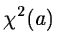to have an extremum is that the partial derivatives vanish i.e.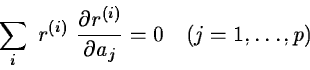or, equivalently,

J(a)T r(a) =0   .

This is usually a system of non-linear equations that, numerically, can be solved using the Newton-Raphson's method also called in the one-dimensional case the tangents method. The Taylor development of the function limited to the first order is taken around some initial guesses of the parameters. The resulting linear system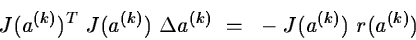gives thus a correction to the solution and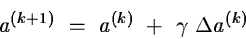is taken as the new approximation of the optimum. The relaxation factoris a parameter of the method. The convergence of the process towards the solution of the non-linear minimization problem has been proven for locally convex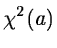or under other assumptions impossible to detail here. These conditions are not generally fulfilled in real problems. Moreover, the algorithm ignores the second order conditions and therefore, may end on a saddle point or never converge. Two different relaxation factors may lead to different solutions or one may give convergence and the other one not. No general rule can be given for the choice of a good relaxation factor.Next: The Modified Gauss-Newton Method. Up: Outline of the Available Previous: Outline of the Available
http://www.eso.org/midas/midas-support.html
1999-06-09